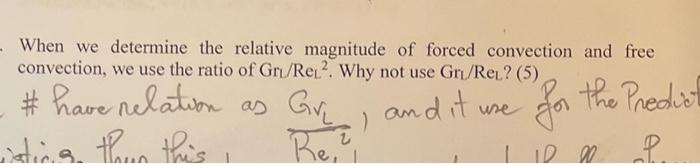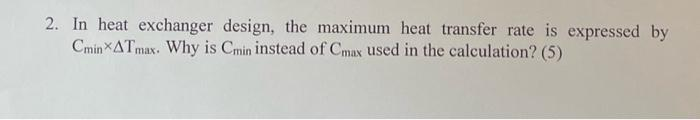Home / Expert Answers / Mechanical Engineering / when-we-determine-the-relative-magnitude-of-forced-convection-and-free-convection-we-use-the-ratio-pa753

# (Solved): When we determine the relative magnitude of forced convection and free convection, we use the ratio ...When we determine the relative magnitude of forced convection and free convection, we use the ratio of . Why not use ? (5) \# have relation as , and it use for the Predic 2. In heat exchanger design, the maximum heat transfer rate is expressed by . Why is instead of used in the calculation? (5)

We have an Answer from Expert